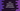# Generators in dart with examples## Dart generators :

Dart generators are used to produce a sequence of values lazily. We have two different types of generators called synchronous and asynchronous generators. In this post, I will show you how these generators works with examples.

### Synchronous generator :

sync* is used to create one synchronous generator function. This is used just before the function body. Synchronous generators returns one Iterable object. For returning a object, yield is used.

For example :

``````Iterable<int> oddNumbersGenerator(n) sync* {
while (n > 0) {
if (n % 2 != 0) {
print("yielding...");
yield n;
}
n--;
}
}
main(List<string> args) {
oddNumbersGenerator(5).forEach(print);
}``````

oddNumbersGenerator is a synchronous generator. It returns one Iterable. It takes one number and for all numbers starting from it to 1, it delivers if it is odd. yield is used to deliver a value.

I have added one print statement before yield is called to help you understand how it works. It will print the below output :

``````yielding...
5
yielding...
3
yielding...
1``````

i.e. each time forEach is called, it generates the next value.

### Asynchronous generator :

asynchronous generators are similar to synchronous generators. The only difference is that we need to use _async* before the function body and it needs to return one Stream. It also uses yield to generate the next value._

We can change the above synchronous generator to asynchronous like below :

``````Stream<int> oddNumbersGenerator(n) async* {
while (n > 0) {
if (n % 2 != 0) {
print("yielding...");
yield n;
}
n--;
}
}
main(List<string> args) {
oddNumbersGenerator(5).forEach(print);
}``````

It will print the same output as the synchronous generator.

### Recursive generator :

We can call one generator function recursively. yield* function is used to call a function recursively. For example, we can change the above example to a recursive generator :

``````Iterable<int> oddNumbersGenerator(n) sync* {
if (n > 0) {
if (n % 2 != 0) {
yield n;
}
yield* oddNumbersGenerator(n - 1);
}
}
main(List<string> args) {
oddNumbersGenerator(5).forEach(print);
}``````

If the value of n is greater than zero, it calls the same function recursively. If the value is not divisible by 2, it returns it.